# IMPLEMENTATION OF HUFFMAN CODING

Huffman coding (or encoding) is a lossless data compression technique based on an algorithm developed by David A. Huffman in 1952. A variable length bit level code is assigned for each character in this technique. The length of the code is related to frequency of characters. Most frequent character is represented by the smallest code while least frequent character gets longest code. Since the frequencies of characters vary for different data sets or files, there is not one code table that efficiently works for every data sets.

Implementation of Huffman algorithm has four main steps;

• determine the frequencies
• apply Greedy Algorithm to build prefix tree
• establish code table
• encode the data

Here is an example of implementing Huffman algorithm in C;

First, variable definitions:

where:

Calculate the frequency of characters;

Build Prefix Tree

• Sort the frequency table
• Take top two (they could be equal to each other)
• Remove them from the table
• Add these two frequencies and place the sum to proper position in the table
• Create a binary tree by using these three numbers
• Label branches of the tree with “1” and “0”. The labelling should be consistent throughout the tree. If left branch is marked with “0”, the right branch should always be “1”.
• Go thru the table and repeat the steps until all items of the table are processed.

example; assume that data set consists of following 10 bytes as input; FF FF 3F 07 15 FF 00 00 00 00

Created binary tree;

 Char Freq Leaf 3F 1 2 07 1 15 1 FF 2 00 4

 Char Freq Leaf 15 1 3 leaf 2 FF 2 00 4

 Char Freq Leaf FF 2 5 leaf 3 00 4

 Char Freq Leaf 00 4 9 leaf 5

Here is the binary tree;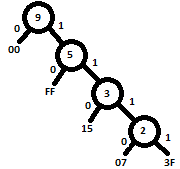Huffman code table;

 Char Freq Code 3F 1 1111 07 1 1110 15 1 110 FF 2 10 00 4 0

Last step is to convert input data to encoded data;

 FF FF 3F 07 15 FF 00 00 00 00 80 bits 10 10 1111 1110 110 10 0 0 0 0 21 bits

Encoded data in hex is; 19 FC A0. Since data can be stored to disks in bytes, the coded data needs to be padded by “0”s at MSB or LSB side. This example uses padding at MSB site. As a result, the input is compressed by 3 times since It takes 3 bytes while the original is 10 bytes.

Hovewer, Huffman code table will be needed to decompress the data. Therefore, it needs to be stored with the compressed data. The size of compressed data file will be increased by the size of the table.

Because of this overhead, size of compressed file could be more than original, if the size of original data is smaller than size of Huffman code table.

Guestbook
 *Name E-mail *Comment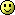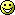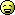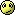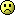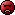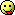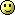Fields marked with an asterisk * are required.
Pages: (0)